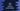# 3 different Java programs to find the next prime number## Java program to find the next prime number:

In this post, we will learn how to find the next prime number in Java. The program will take one number as its input and it will find the next prime number starting from that number.

The program will use one function to check if a number is a prime number or not. We can use a loop to iterate over the numbers starting from the given number and for each number, the program will check for the prime number. Once a prime number is found, it will print that number and exit from the loop.

### What is a prime number:

A number is called a prime number if the number is greater than 1 and it is divisible only by 1 and the number itself.

For example, 23 is a prime number. Because there is no number other than 1 and 23 that can divide it.

Similarly, 20, 21, 22 etc. are not prime numbers.

### Example 1: Java program to find the next prime number:

Let’s take a look at the below example program:

``````import java.util.Scanner;

public class Main {

private static boolean isPrime(int no) {
for (int i = 2; i < no; i++) {
if (no % i == 0) {
return false;
}
}
return true;
}

private static int getNextPrime(int no) {
for (int i = no + 1; ; i++) {
if (isPrime(i)) {
return i;
}
}
}

public static void main(String[] args) {
Scanner scanner = new Scanner(System.in);
int no;

System.out.println("Enter a number: ");
no = scanner.nextInt();

System.out.println("Next prime is: " + getNextPrime(no));
}
}``````
• This Java program finds the next prime number. It reads the user input number and assigns it to the no variable.
• The getNextPrime function finds the next prime number.
• It uses a for loop that runs from one number after the given number and on each iteration, it checks if the current number is prime or not.
• To check for a prime number, we are using the isPrime method.
• This method uses a for loop to run from i = 2 to i = number - 1 and if any value of i can divide the number, it returns false. Else, it returns true.

It prints output as below:

### Example 2: By iterating up to half of the number:

We can improve the prime number checking method to run the loop from 2 to number/2 as all other numbers larger than number/2 can’t divide the number. It will reduce the loop iteration.

``````import java.util.Scanner;

public class Main {

private static boolean isPrime(int no) {
for (int i = 2; i <= no/2; i++) {
if (no % i == 0) {
return false;
}
}
return true;
}

private static int getNextPrime(int no) {
for (int i = no + 1; ; i++) {
if (isPrime(i)) {
return i;
}
}
}

public static void main(String[] args) {
Scanner scanner = new Scanner(System.in);
int no;

System.out.println("Enter a number: ");
no = scanner.nextInt();

System.out.println("Next prime is: " + getNextPrime(no));
}
}``````

The only change in the program is the for loop of isPrime method. It will give similar results.

``````Enter a number:
20
Next prime is: 23

Enter a number:
100
Next prime is: 101

Enter a number:
101
Next prime is: 103``````

### Example 3: By iterating up to the square root of the number:

We can further optimize the above program by iterating up to the square root of the number. The loop will run from 2 to square root of the number.

``````import java.util.Scanner;

public class Main {

private static boolean isPrime(int no) {
for (int i = 2; i <= Math.sqrt(no); i++) {
if (no % i == 0) {
return false;
}
}
return true;
}

private static int getNextPrime(int no) {
for (int i = no + 1; ; i++) {
if (isPrime(i)) {
return i;
}
}
}

public static void main(String[] args) {
Scanner scanner = new Scanner(System.in);
int no;

System.out.println("Enter a number: ");
no = scanner.nextInt();

System.out.println("Next prime is: " + getNextPrime(no));
}
}``````

We are using Math.sqrt method to find the square root. It gives similar output.